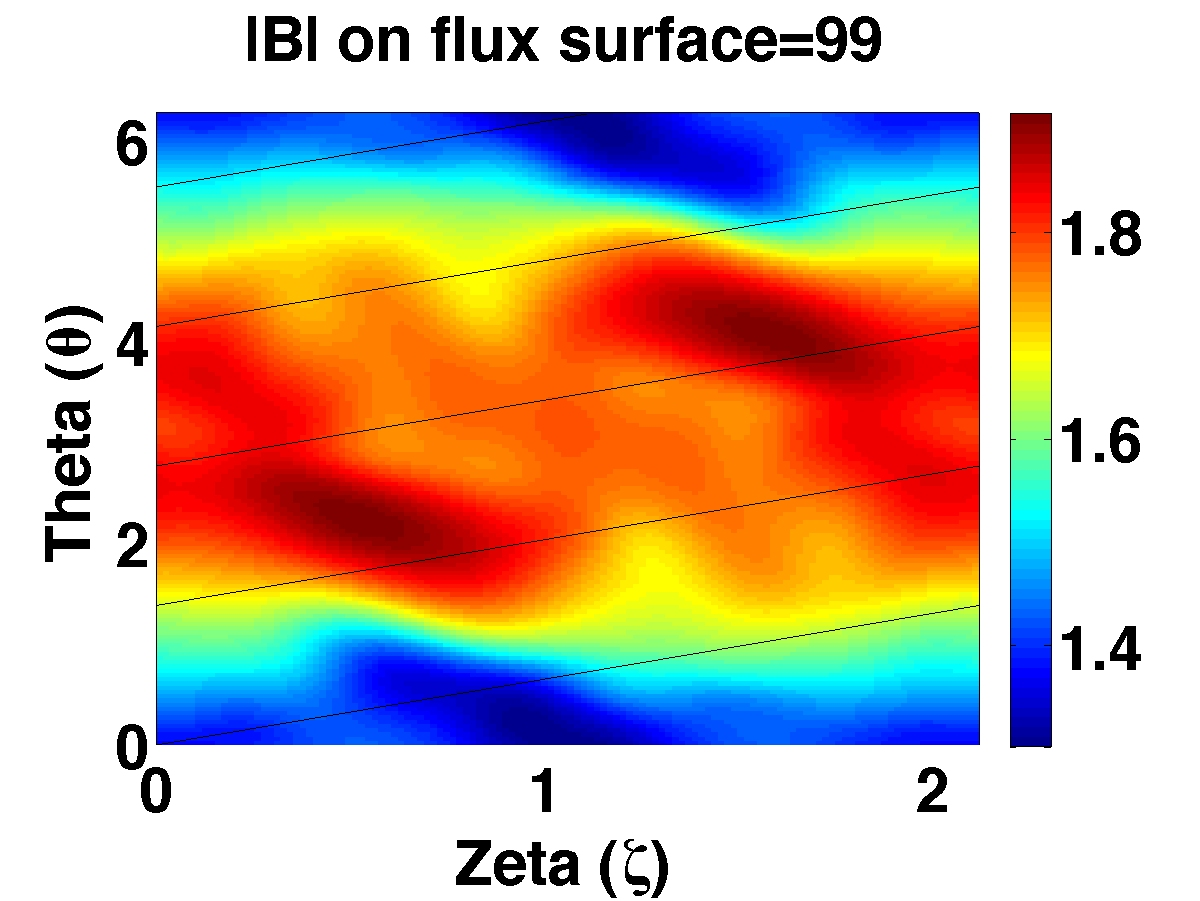STELLOPT

State-of-the-art stellarator optimization code

BOOZ_XFORM

This code calculates the transformation to Boozer coordinates (Boozer A. H. "Plasma equilibrium with rational magnetic surfaces." Phys. Fluids 24, 1999 (1981)). of a VMEC equilibria.Theory

The BOOZ_XFORM code Sanchez R., Hirshman S.P., Ware A. S., Berry L. A., and Spong D.A. "Ballooning stability optimization of low-aspect-ratio stellarators." Plas. Phys. Cont. Fusion 42, 641 (2000). transforms the VMEC field-line coordinate system to the straight field-line coordinates introduced by Boozer (Fusionwiki link). The Boozer coordinates allow the magnetic field to be written in the form

$$\vec{B}=\nabla\psi\left(s\right)\times\nabla\zeta_b+\nabla\theta_b\times\nabla\chi\left(s\right)$$

where the subscript indicates the quantities are generalized toroidal and poloidal coordinates (xi and theta respectively). The code transforms the coordinates and magnetic field. The output quantities are in terms of the Fourier coefficients of the transformed quantities. It is important to note that Boozer coordinates may require many more Fourier modes than the equivalent VMEC representation to maintain accuracy. In general, it is useful to choose between 5 and 6 times more poloidal and 2-3 times more toroidal Fourier resolution than those found in the VMEC equilibria.

Note: As of v6.9 only stellarator symmetric equilibria can be transformed. The version distributed with VMEC 8.47 can handle non-stellarator symmetric equilibria.

Compilation

BOOZ_XFORM is a component of the STELLOPT suite of codes. Compilation of the STELLOPT suite is discussed on the STELLOPT Compilation Page.

Input Data Format

The BOOZ_XFORM code takes an input filename as a command line argument. The input file contains the number of theta and zeta harmonics to use, the filename extension of the wout file to read, and a list of surfaces on which to calculate the transform. Typically the user will choose surfaces located at 1/4, 1/2, and 3/4 the way from the axis to the edge of the equilibrium. The choice of surface must coincide with a VMEC index. For example, if the user wished to specify 72 poloidal harmonics, 15 toroidal harmonics, the ‘wout.test’ file, and surfaces 25, 50 and 75, the file would look like:

72  15
'test'
25 50 75

Execution

The BOOZ_XFORM code takes a command line (see above) and optional output suppression arguments (T/F). For example, if the user had an input file entitled ‘in_booz.test’ the code could be executed by:

> xbooz_xform in_booz.test
0 <= mboz <=   71    -15 <= nboz <=   15
nu_boz =   290 nv_boz =    62

OUTBOARD (u=0)              JS          INBOARD (u=pi)
-----------------------------------------------------------------------------
v     |B|vmec    |B|booz    Error             |B|vmec    |B|booz    Error

0    1.452E+00  1.452E+00  2.350E-10   25    1.571E+00  1.571E+00  2.792E-10
pi    1.455E+00  1.455E+00  4.914E-10         1.560E+00  1.560E+00  6.248E-11
0    1.383E+00  1.383E+00  2.688E-09   50    1.688E+00  1.688E+00  2.026E-07
pi    1.364E+00  1.364E+00  3.235E-09         1.657E+00  1.657E+00  5.820E-07
0    1.378E+00  1.378E+00  1.047E-06   75    1.796E+00  1.796E+00  2.146E-06
pi    1.307E+00  1.307E+00  7.323E-07         1.784E+00  1.784E+00  5.163E-06

TIME IN BOOZER TRANSFORM CODE:    3.02E+00 SEC

Output Data Format

The transformed R, Z, p and |B| quantities are output into a file ‘boozmn.ext’ where ext is the VMEC file extension specified in the input file. The STELLOPT library provides a fortran function for reading this file: read_boozer_file.f. This file can be included in a code through read_booz_mod. The output file is a binary file a general prescription for reading the file can be found below (taken from read_boozer_file):

read(iunit, iostat=ierr, err=100) nfp_b, ns_b, aspect_b, max_b, rmin_b, betaxis_b

do nsval = 2, ns_b
read(iunit, iostat=ierr, err=100) iota_b(nsval), pres_b(nsval), beta_b(nsval), phip_b(nsval), phi_b(nsval), bvco_b(nsval), buco_b(nsval)
end do

read(iunit, iostat=ierr, err=100) mboz_b, nboz_b, mnboz_b

do mn = 1, mnboz_b
end do

do mn = 1, mnboz_b
read(iunit, iostat=ierr, err=100, end=200) bmn_b(mn,nsval), rmnc_b(mn,nsval), zmns_b(mn,nsval), pmns_b(mn,nsval), gmn_b(mn,nsval)
end do

do while (ierr .eq. 0)
do mn = 1, mnboz_b
read(iunit, iostat=ierr, err=100, end=200) bmn_b(mn,nsval), rmnc_b(mn,nsval), zmns_b(mn,nsval), pmns_b(mn,nsval), gmn_b(mn,nsval)
end do
end do

Note that this is for version v1.0. The latest version v2.0 outputs in netCDF format.

Visualization

Once read from the output file the BOOZ_XFORM data can be transformed to real space much the way VMEC data is. It is important to note that this code utilizes a trigonometric argument of the form (mu-nv). In Boozer coordinates the toroidal angle is optimized so before preforming a coordinate transformation the user should first transform the pmns (sin function) and add the values to the toroidal angle. This angle should then be used for transforming the remaining quantities.

Tutorials

Boozer Transformation for NCSX-Like Configuration

Explanation of the VMEC to Boozer coordinate transformation